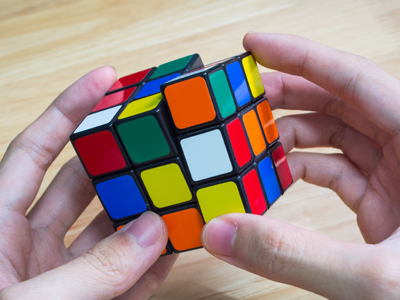If you find cubes confusing, then this quiz will help!

# Perimeter and Area 3 (Difficult)

So, you have made it to the third in our difficult series of Eleven Plus maths quizzes on perimeter and area – give yourself a pat on the back for making it this far! In your exam you will have to work out the perimeter and area of 2D shapes, like squares, along with the surface area and volume of 3D shapes, like cubes. This quiz will help you practice.

While we are on the subject of shapes, here are the names of some lesser-known ones:

• Triangle: 3 sides
• Pentagon: 5 sides
• Hexagon: 6 sides
• Heptagon: 7 sides
• Octagon: 8 sides
• Nonagon: 9 sides
• Decagon: 10 sides
• Hendecagon: 11 sides
• Dodecagon: 12 sides

Don’t worry – you won’t need to know all of these - but if you can remember them all, they may come in useful at some point.

1.
What do we call the longest side of a right-angled triangle?
The scalene
The isosceles
The hypotenuse
The equilateral
It’s the side opposite the right-angle
2.
A cuboid has a height of 5 cm. What is its surface area?
150 cm2
125 cm2
100 cm2
Not enough information to answer the question
The surface area of a cube = height x height x 6 (because it has 6 faces). However, the surface area of a cuboid is length x height x 6. We do not know the length of this cuboid, so we cannot answer the question.
Make sure you don’t confuse cuboids with cubes!
3.
What is the area of a circle whose diameter = 20 cm (take pi to be 3.142)?
1,256.8 cm2
314.2 cm2
125.6 cm2
31.42 cm2
The formula for the area of a circle is πr2. As you know, the radius is half the diameter, so the area of this circle will be:
3.142 x (20 ÷ 2)2 =
3.142 x 102
3.142 x 100 = 314.2 cm2
4.
Square A has an area of 25 cm2. Square B is twice as wide as square A. What is the area of square B?
50 cm2
75 cm2
100 cm2
125 cm2
If you double the width of a square, its area quadruples (times by 4). Here’s the proof:
Square A: 5 x 5 = 25
Square B: 10 x 10 = 100
5.
A cube has a height of 2 cm. What is its volume?
24 cm3
8 cm2
8 cm3
6 cm3
The volume of a cube = length3:
2 x 2 x 2 = 8 cm3.
Make sure you don’t confuse cubed with squared!
6.
Circle A has a radius of 10 cm and a circumference of 63 cm. If it is cut into two equal sized semicircles, what is the circumference of one of those semicircles?
51.5 cm
41.5 cm
31.5 cm
21.5 cm
If you cut a circle into two semicircles their circumference will be half the circle’s circumference + the circle’s diameter (radius x 2).
(63 ÷ 2) + (10 x 2) = 31.5 + 20 = 51.5 cm
7.
The height of a cube is 4 cm. What is its total surface area?
32 cm2
64 cm2
96 cm2
3D shapes do not have area
3D shapes do have an area, which we call 'surface area'.
A cube has six faces, each of which is a square, so to find its surface are we work out the area of one face and then multiply by 6
4 x 4 x 6 = 96 cm2
8.
Circle A has an area of 64 cm2. Circle B has a radius a quarter the size of Circle A’s radius. What is the area of circle B?
16 cm2
24 cm2
32 cm2
48 cm2
If you double the area of a circle, the radius is decreased fourfold (divided by 4). Conversely, if you decrease the radius of a circle by dividing it by 4, the area is halved
9.
A right-angle triangle has a base of 16 cm and a height of 12 cm. What is its area?
192 cm2
96 cm2
146 cm2
73 cm2
The area of a right-angle triangle = (base × height) ÷ 2.
(16 x 12) ÷ 2 = 192 ÷ 2 = 96 cm2
10.
A regular decagon has sides measuring 7 cm each. What is its perimeter?
70 cm
63 cm
56 cm
49 cm
The length of the perimeter of a regular polygon is found by multiplying the length of one of its sides by the number of sides. Decagons have ten sides, so 7 x 10 = 70 cm
Author:  Frank Evans